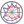Course title
Mechanics of Materials Exercises
 hashimura shinjiCourse description
When mechanical engineers design various mechanical structures and investigate accident causes, they have to always use knowledge with regard to Mechanics of Materials. Hence it is very important to solve various practice exercises based on actual structures to learn Material Mechanics.
In this course, students solve the various practical exercises with regard to Mechanics of Materials, which are prepared, everytime. Answers and ways to solve these problems are also explained.
Purpose of class
The subject of the lecture is that students can solve any problems with regard to Mechanics of Materials. And the students can also model actual structures and machines to enable to solve by means of Mechanics of Materials theoretically.
Goals and objectives
1. To calculate displacements of truss structures which are receiving loads.
2. To calculate twisting angle of circular bar which is receiving loads.
3. To calculate deflection and deflection angle of beams which are receiving loads.
4. To calculate deformations and stresses of beams which are receiving combined stress.
5. To calculate deformations and stresses of complex structures which are receiving loads.
Language
English
Class schedule

Class schedule HW assignments (Including preparation and review of the class.) Amount of Time Required
1. Guidance
Elongations of a bar which received tension and compression
Prepare how to calculate elongations and shrinkages in tension-compression problems 90minutes
Review of how to calculate elongations and shrinkages in tension-compression problems 100minutes
2. Deformation of truss structures Prepare how to calculate deformation of truss structures 90minutes
Review of how to calculate deformation of truss structures 100minutes
3. Strain energy method in tension-compression problems Prepare how to calculate elongations and shrinkages in tension-compression problems using the strain energy method 90minutes
Review of how to calculate elongations and shrinkages in tension-compression problems using the strain energy method 100minutes
4. Exercises for tension-compression problems Prepare how to calculate displacements in tension-compression problems 90minutes
Solve exercises for tension-compression problems 100minutes
5. Shearing stress and polar moment of inertia of circular bars Prepare how to calculate shearing stress and polar moment of inertia of circular bars 90minutes
Review of how to calculate shearing stress and polar moment of inertia of circular bars 100minutes
6. Twisting angle of circular bars and exercises for torsion problems Prepare how to calculate twisting angle of circular bars and exercises for torsion problems 90minutes
Review of how to calculate twisting angle of circular bars and exercises for torsion problems 100minutes
7. Quiz
Shearing force diagrams and bending moment diagrams of beams
Prepare how to draw shearing force diagrams and bending moment diagrams of beams 90minutes
Review of how to draw shearing force diagrams and bending moment diagrams of beams 100minutes
8. Deflection for beams subjecting to bending moment Prepare how to calculate deflection for beams subjecting to bending moment 90minutes
Review of how to calculate deflection for beams subjecting to bending moment 100minutes
9. Strain energy method to calculate deflection for beams subjecting to bending moment Prepare how to calculate deflection for beams subjecting to bending moment using the strain energy method 90minutes
Review of how to calculate deflection for beams subjecting to bending moment using the strain energy method 100minutes
10. Exercises to calculate deflection for beams subjecting to bending moment Prepare how to calculate deflection of beams subjecting to bending moment 90minutes
Solve exercises for deflection of beams subjecting to bending moment 100minutes
11. Displacement of a beam subjecting to tension-compression, twisting torque and bending moment Prepare how to calculate Displacement of a beam subjecting to tension-compression, twisting torque and bending moment 90minutes
Review of how to calculate Displacement of a beam subjecting to tension-compression, twisting torque and bending moment 100minutes
12. Exercises to calculate displacement of a beam subjecting to tension-compression, twisting torque and bending moment Prepare how to calculate displacement of a beam subjecting to tension-compression, twisting torque and bending moment 90minutes
Solve exercises for displacement of a beam subjecting to tension-compression, twisting torque and bending moment 100minutes
13. Stress of a beam subjecting to tension-compression, twisting torque and bending moment Prepare how to calculate stress of a beam subjecting to tension-compression, twisting torque and bending moment 90minutes
Review of how to calculate stress of a beam subjecting to tension-compression, twisting torque and bending moment 100minutes
14. Stress of a beam subjecting to tension-compression, twisting torque and bending moment
Quiz
Prepare how to solve all problem that we did 90minutes
Confirm answers of the examination 100minutes
Total. - - 2660minutes
Relationship between 'Goals and Objectives' and 'Course Outcomes'

report Quiz Total.
1. 10% 8% 18%
2. 10% 8% 18%
3. 10% 8% 18%
4. 15% 8% 23%
5. 15% 8% 23%
Total. 60% 40% -
Evaluation method and criteria
Quiz:40%, Reports: 60%
Students must submit all reports given.
Students are going to pass by submitting all reports and solving 40 % problems with the example level.
Students can take A score by submitting all reports and solving 60 % problems with the example level.
Students can take S score by submitting all reports and solving 100 % problems with the example level.
Textbooks and reference materials
Prints mainly, Text book of Strength of materials
Prerequisites
You should learn mechanics of materials in advance.
Office hours and How to contact professors for questions
• Lunch time on Friday.